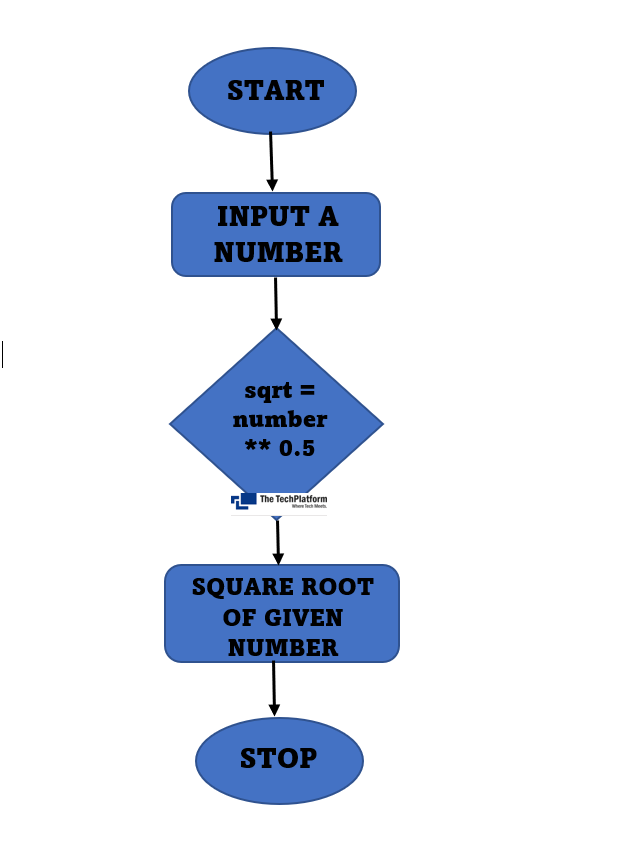top of page
Search

# Program to Square a Number in PythonIn this, we will learn 3 different ways to find the Square root of a number in Python

1. Using Exponent

2. Using math.sqrt()

3. Using math.pow()

#### Flowchart### Method 1: Using Exponent

Here we are using the exponent (**) sign, which is used to find out the power of a number.

Code:

```number = int(input("Enter a Number: "))
sqrt = number ** 0.5
print("Square Root of given Number is:", sqrt)```

Output:### Method 2: Using math.sqrt()

sqrt() is the predefined method used to find square root in python. But we have to import the math module to use the sqrt() method.

Code:

```import math
number = int(input("Enter a Number:"))
sqrt = math.sqrt(number)
print("Square Root of given Number:" , sqrt)```

Output:### Method 3: Using math.pow()

pow() is also a predefined method to find out the power of a number, it takes two arguments as input, the first is the number itself and the second one is the power of that number.

Code:

```import math
number = int(input("Enter a Number:"))
sqrt = math.pow(number, 0.5)
print("Square Root of given Number: ", sqrt)```

Output:The Tech Platform